# Naming segments, rays, and lines

Point − A basic geometric entity. It that has no length, width or height. It is described only by its position.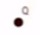This is a point Q

Straight line − A set of points that extend indefinitely in both directions.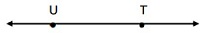This is an example of line UT.

A line is a continuous set of points that continues on indefinitely. The arrow indicates infinite length.

Line segment − A part of a line with two end points say X and Y.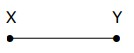This is an example of line segment XY.

Ray − A part of a line with one endpoint. A ray extends indefinitely in one direction.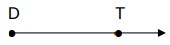This is an example of ray DT.

Identify given figure as a line segment, a ray or a line. Then name the figure using the given points.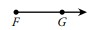### Solution

Step 1:

The given figure has one end point and continues indefinitely in one direction. Therefore, it is a ray.

Step 2:

The ray is named as FG.

Identify given figure as a line segment, a ray or a line. Then name the figure using the given points.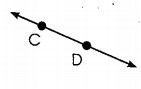### Solution

Step 1:

The given figure continues indefinitely in both directions. So it is a line.

Step 2:

The line is named as CD.